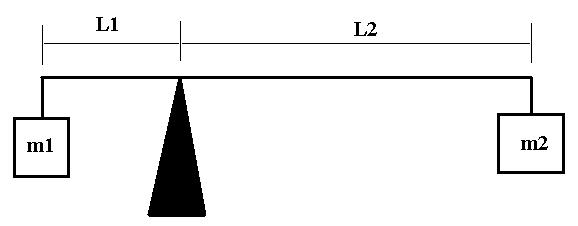# Pivot point of a beam and torque

## Homework Statement

A beam is supported only at one point, called the pivot point, as shown in the diagram. A block with mass m1 sits at the left end of the beam, a distance L1 from the pivot point. A block with mass m2 sits at the right end of the beam, a distance L2 from the pivot point. L2 > L1. Calculate all torques about the pivot point, remembering that positive is anti-clockwise.
Select Yes, No, Less than, Equal to, or Cannot tell.

• Given particular values of L1, L2, and m1, is it always possible to choose m2 such that the masses have no angular acceleration?
• If m1 * L2 = m2 * L1, is there a negative torque?
• For m1 = m2, does the angular acceleration depend only on L1 / L2 ? (If it depends on the actual values of L1 and L2, put 'no'.)
• If m1 * L1 = m2 * L2, will the masses have an angular acceleration?

Torque = Force*L

## The Attempt at a Solution

• For the first statement, since L2 is always greater than L1 by a certain ratio, if M2 is less than M1 by that same ratio, then there would be no torque, and thus no angular acceleration. However, I'm not certain my thought process is correct.
• Since the two lengths are flipped, and no exact masses are given, there is no way to determine if this statement is true or false.
• I'm really stuck on this one.... I put no, since the torque is calculated from the sum of the each individual torque, not the product nor the quotient.
• If the two torques are the same, then the beam wouldn't move, and thus would have no angular acceleration.

ehild
Homework Helper

## Homework Statement

A beam is supported only at one point, called the pivot point, as shown in the diagram.

The diagram is not show, attach it, please!

ehildhere is the attached image that came with the problem.

Last edited:
ehild
Homework Helper
[*]For m1 = m2, does the angular acceleration depend only on L1 / L2 ? (If it depends on the actual values of L1 and L2, put 'no'.)

## The Attempt at a Solution

• I'm really stuck on this one.... I put no, since the torque is calculated from the sum of the each individual torque, not the product nor the quotient.

• "NO" is the correct answer. You know that the angular acceleration is torque/moment of inertia. The moment of inertia is m1L12+m2L2 in this case.

The angular acceleration is
$$\alpha=g \frac{m_1L_1-m_2L_2}{m_1L_1^2+m_2L_2^2}$$.
When the masses are equal they cancel, and the angular acceleration becomes
$$\alpha=g\frac{L_1-L_2}{L_1^2+L_2^2}=g\frac{L_1/L_2-1}{L_2(1+(L_1/L_2)^2)}$$

it depends on the actual lengths, not only on their ratio.

ehild

Sweet, thanks, could you possibly help determine if my answers to the other statements are right?

I gave "yes" to the first statement, cannot tell to the second and no to the fourth... but it is not correct...

ehild
Homework Helper
Oops, I forgot to say that the other answers were correct. There were no problems with them.

ehild

I've already tried yes, cannot tell, no, no for the four statements... however, the online homework set still says that my answers are incorrect.

ehild
Homework Helper
For the second question, I just noticed that L2>L1 was given. You can find out if the torque is negative or not.
It says that m1L2=m2L1, so m2=m1(L2/L1).

Substitute for m2 in the equation for the torque and see what you get with the condition L2>L1.

ehild

Could you please see if the following is correct?

Torque1 = m1*L1
Torque2 = m1*(L2)2/L1

Since L2 is greater than L1, then Torque2 is greater in magnitude than Torque1, and since counterclockwise is positive, there is negative torque.

ehild
Homework Helper
YES!!!

ehild

Sweet thank you so much!

ehild
Homework Helper
And a sweet welcome to you!ehild